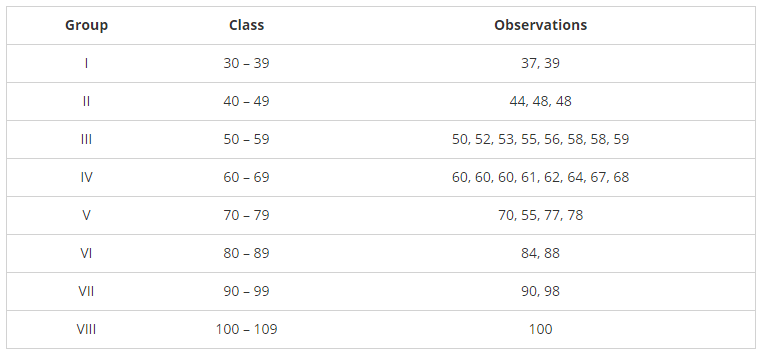# The final marks in mathematics of 30 students are as follows:

Question:

The final marks in mathematics of 30 students are as follows:

53, 61, 48, 60, 78, 68, 55, 100, 67, 0, 75, 88, 77, 37, 84, 58, 60, 48, 62, 56, 44, 58, 52, 64, 98, 59, 70, 39, 50, 60.

(i) Arrange these marks in ascending order 30 to 39 one group 40 to 49 second group etc.

(ii) What is the lowest score?

(iii) What is the highest score?

(iv) What is the range?

(v) If 40 is the pass mark how many failed?

(vi) How many have scored 75 or more?

(vii) Which observations between 50 and 60 have not actually appeared?

(viii) How many have scored less than 50?

Solution:

The final marks in mathematics of 30 students are as follows:

53, 61, 48, 60, 78, 68, 55, 100, 67, 0, 75, 88, 77, 37, 84, 58, 60, 48, 62, 56, 44, 58, 52, 64, 98, 59, 70, 39, 50, 60.

(i)(2) Highest score = 100

(3) Lowest score = 37

(4) Range = 100 - 37 = 63

(5) If 40 is the passing marks 2 students have failed

(6) 8 students have scored 75 or more

(7) Observation 51, 54, 57 between 50 and 60 has not actually appeared.

(8) 5 students have scored less than 50